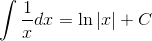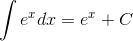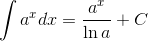### 4.A. Antiderivatives

 Antiderivatives are simply the inverse of derivatives.This section will focus on the antiderivatives of power, exponential, and logarithmic functions.Key Termsindefinite integralantidifferentiationantiderivativeexponential functionlogarithmic functionEssential QuestionsHow can antiderivatives be found using derivative rules?Exponential and Logarithmic Derivatives and Antiderivatives